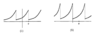# Fourier series for a series of functions

EnriqueOrtizMartinez
Homework Statement:
From equations 1) or 2) use the appropriate values of ## \theta ## usually ## (0 ,\frac{\pi }{2},\pi )## , to get to the indicated equation.
Relevant Equations:
1)

## f(\theta )=e^{b\theta }(-\pi <\theta <\pi ) \quad | \quad\frac{sinh(b\pi )}{\pi }\sum_{-\infty }^{\infty }\frac{(-1)^{n}}{b-in}e^{in\theta } ##

2)

##f(\theta )=e^{b\theta }(0<\theta <2\pi )\quad | \quad\frac{e^{2\pi b}-1}{2\pi }\sum_{-\infty }^{\infty }\frac{e^{in\theta }}{b-in}##

Indicated equation:

##\sum_{1 }^{\infty }\frac{(-1)^{n}}{n^{2}+b^{2}}=\frac{\pi }{2b}csch(b\pi )-\frac{1}{2b^{2}}##
## ##

## e^{b\theta }=\frac{sinh(b\pi )}{\pi }\sum_{-\infty }^{\infty }\frac{(-1)^{n}}{b-in}e^{in\theta } ##

If ## \theta =0 ##

##e^{b(0)}=\frac{sinh(b\pi )}{\pi }\sum_{-\infty }^{\infty }\frac{(-1)^{n}}{b-in}e^{in(0) }##

##1=\frac{sinh(b\pi )}{\pi }\sum_{-\infty }^{\infty }\frac{(-1)^{n}}{b-in} ##

Using the conjugate of the complex:

##1=\frac{sinh(b\pi )}{\pi }\sum_{-\infty }^{\infty }\frac{(-1)^{n}}{b-in}\frac{b+in}{b+in} ##

## 1=\frac{sinh(b\pi )}{\pi }\sum_{-\infty }^{\infty }(-1)^{n}\frac{b+in}{b^{2}+n^{2}}##

## \pi csch(b\pi )=\sum_{-\infty }^{\infty }(-1)^{n}\frac{b+in}{b^{2}+n^{2}}##

Up to now I have only arrived, I do not know if it is for equation 1 to arrive at the result or for equation 2, another doubt that I have is that the summations of equations 1) and 2) tend to ##(-\infty ,\infty ) ## and the result of the summation tends to ## (1,\infty )## , as I do so that the sum of ## (-\infty ,\infty )## tend a ## (1,\infty )## ,my idea was to see if the functions were even or odd but since both are exponential they are not odd or even, they can help me with my problem in advance thanks.

#### Attachments

•2.-Fourier.png
7.8 KB · Views: 249

The terms for $n > 0$ and $-n$ are complex conjugates. This reordering of terms is justifiable if you treat the summation as $$\lim_{N \to \infty} \sum_{n = -N}^{N} (-1)^n \frac{b + in}{b^2 + n^2}.$$